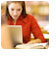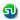### Simple and compound interest practice questions, answers. CAT, XAT, TANCET, PGSEM, MAT MBA Entrance Test Preparation

 Home TANCET Classes GMAT Coaching Online GMAT Course CAT Classes TANCET Study Material
 TANCET '18 Classroom ProgramWeekend and weekday classes for TANCET MBA @ Chennai. At Nungambakkam and Velachery.Other Courses TANCET Correspondence Course CAT Classes & Courses GMAT Classes Chennai Online GMAT Course GRE Classes Chennai CBSE Math Online Tuition SAT Classes Other Links Ascent TANCET Toppers Testimonials CAT, TANCET Questions Careers @ Ascent Contact Us +91 44 4500 8484 +91 96000 48484 ascent@ascenteducation.com Postal Address Facebook / Twitter / Blog / Videos
You are here: Home  »  CAT, XAT, TANCET, Sample Questions  »  Simple & Compound Interest

# Simple & Compound Interest Questions, Answers

###### Simple Interest, Compound Interest, Annuity, EMIs, Time taken to double money
A collection of questions that typically appear from the topic simple and compound interest in Indian Business Schools entrance tests. An easy topic to master. You could expect one to two questions from this topic.
1. Question 1 »  A father left a will of Rs.35 lakhs between his two daughters aged 8.5 and 16 such that they may get equal amounts when each of them reach the age of 21 years. The original amount of Rs.35 lakhs has been instructed to be invested at 10% p.a. simple interest. How much did the elder daughter get at the time of the will? Explanatory Answer »

2. Question 2 »  What will Rs.1500 amount to in three years if it is invested in 20% p.a. compound interest, interest being compounded annually? Explanatory Answer »

3. Question 3 »  If a sum of money grows to 144/121 times when invested for two years in a scheme where interest is compounded annually, how long will the same sum of money take to treble if invested at the same rate of interest in a scheme where interest is computed using simple interest method? Explanatory Answer »

4. Question 4 »  The population of a town was 3600 three years back. It is 4800 right now. What will be the population three years down the line, if the rate of growth of population has been constant over the years and has been compounding annually? Explanatory Answer »

5. Question 5 »  A man invests Rs.5000 for 3 years at 5% p.a. compound interest reckoned yearly. Income tax at the rate of 20% on the interest earned is deducted at the end of each year. Find the amount at the end of the third year. Explanatory Answer »

6. Question 6 »  The difference between the compound interest and the simple interest on a certain sum at 12% p.a. for two years is Rs.90. What will be the value of the amount at the end of 3 years? Explanatory Answer »

7. Question 7 »  A stairway 10ft high is such that each step accounts for half a foot upward and one-foot forward. What distance will an ant travel if it starts from ground level to reach the top of the stairway? Explanatory Answer »

8. Question 8 »  A sum of money invested for a certain number of years at 8% p.a. simple interest grows to Rs.180. The same sum of money invested for the same number of years at 4% p.a. simple interest grows to Rs.120 only. For how many years was the sum invested? Explanatory Answer »

9. Question 9 »  How long will it take for a sum of money to grow from Rs.1250 to Rs.10,000, if it is invested at 12.5% p.a simple interest? Explanatory Answer »

10. Question 10 »  Rs. 5887 is divided between Shyam and Ram, such that Shyam's share at the end of 9 years is equal to Ram's share at the end of 11 years, compounded annually at the rate of 5%. Find the share of Shyam.Explanatory Answer »

11. Question 11 »  The question for the day is from the topic simple and compound interest. Shawn invested one half of his savings in a bond that paid simple interest for 2 years and received Rs.550 as interest. He invested the remaining in a bond that paid compound interest, interest being compounded annually, for the same 2 years at the same rate of interest and received Rs.605 as interest. What was the value of his total savings before investing in these two bonds? Explanatory Answer »

12. Question 12 »  Rs.100 doubled in 5 years when compounded annually. How many more years will it take to get another Rs.200 compound interest? Explanatory Answer »

13. Question 13 »  In the beginning of the year 2004, a person invests some amount in a bank. In the beginning of 2007, the accumulated interest is Rs.10,000 and in the beginning of 2010, the accumulated interest becomes Rs.25,000. The interest rate is compounded annually and the annual interest rate is fixed. The principal amount is _____: Explanatory Answer »

## CAT, XAT, TANCET Practice Questions and Answers : Listed Topicwise

 Number Theory Permutation Combination Probability Inequalities Geometry Mensuration Trigonometry Coordinate Geometry Percentages Profit Loss Ratio Proportion Mixtures Alligation Speed Time Distance Pipes Cisterns Interest Races Average, Mean AP, GP, HP Set Theory Clocks Calendars Algebra Function English Grammar General Awareness Data sufficiency TANCET Papers XAT PapersAdd to del.icio.usStumble Itdigg this